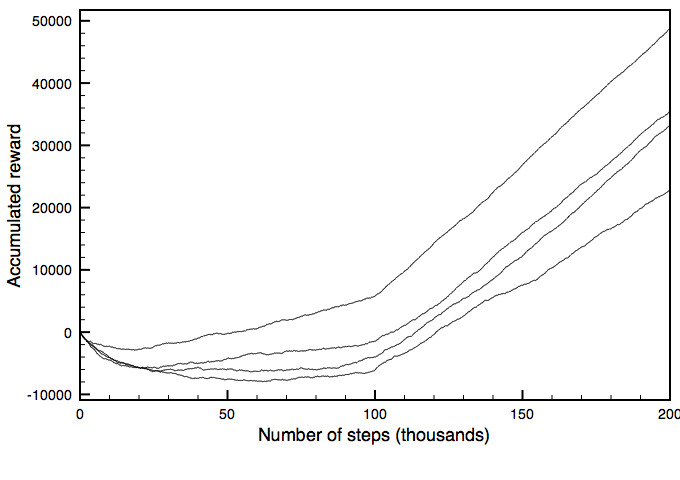### 11.3.5 Evaluating Reinforcement Learning Algorithms

We can judge a reinforcement learning algorithm by how good a policy it finds and how much reward it receives while acting in the world. Which is more important depends on how the agent will be deployed. If there is sufficient time for the agent to learn safely before it is deployed, the final policy may be the most important. If the agent has to learn while being deployed, it may never get to the stage where it has learned the optimal policy, and the reward it receives while learning may be what the agent wants to maximize.Figure 11.12: Cumulative reward as a function of the number of steps

One way to show the performance of a reinforcement learning algorithm is to plot the cumulative reward (the sum of all rewards received so far) as a function of the number of steps. One algorithm dominates another if its plot is consistently above the other.

Example 11.11: Figure 11.12 compares four runs of Q-learning on the game of Example 11.8. These plots were generated using the "trace on console" of the applet available on the course web site and then plotting the resulting data.

The plots are for different runs that varied according to whether α was fixed, according to the initial values of the Q-function, and according to the randomness in the action selection. They all used greedy exploit of 80% (i.e., ε=0.2) for the first 100,000 steps, and 100% (i.e., ε=0.0) for the next 100,000 steps. The top plot dominated the others.

There is a great deal variability of each algorithm on different runs, so to actually compare these algorithms one must run the same algorithm multiple times. For this domain, the cumulative rewards depend on whether the agent learns to visit the repair station, which it does not always learn. The cumulative reward therefore tends to be bimodal for this example. See Exercise 11.8.

There are three statistics of this plot that are important:

• The asymptotic slope shows how good the policy is after the algorithm has stabilized.
• The minimum of the curve shows how much reward must be sacrificed before it starts to improve.
• The zero crossing shows how long it takes until the algorithm has recouped its cost of learning.

The last two statistics are applicable when both positive and negative rewards are available and having these balanced is reasonable behavior. For other cases, the cumulative reward should be compared with reasonable behavior that is appropriate for the domain; see Exercise 11.7.

One thing that should be noted about the cumulative reward plot is that it measures total reward, yet the algorithms optimize discounted reward at each step. In general, you should optimize for, and evaluate your algorithm using, the optimality criterion that is most appropriate for the domain.